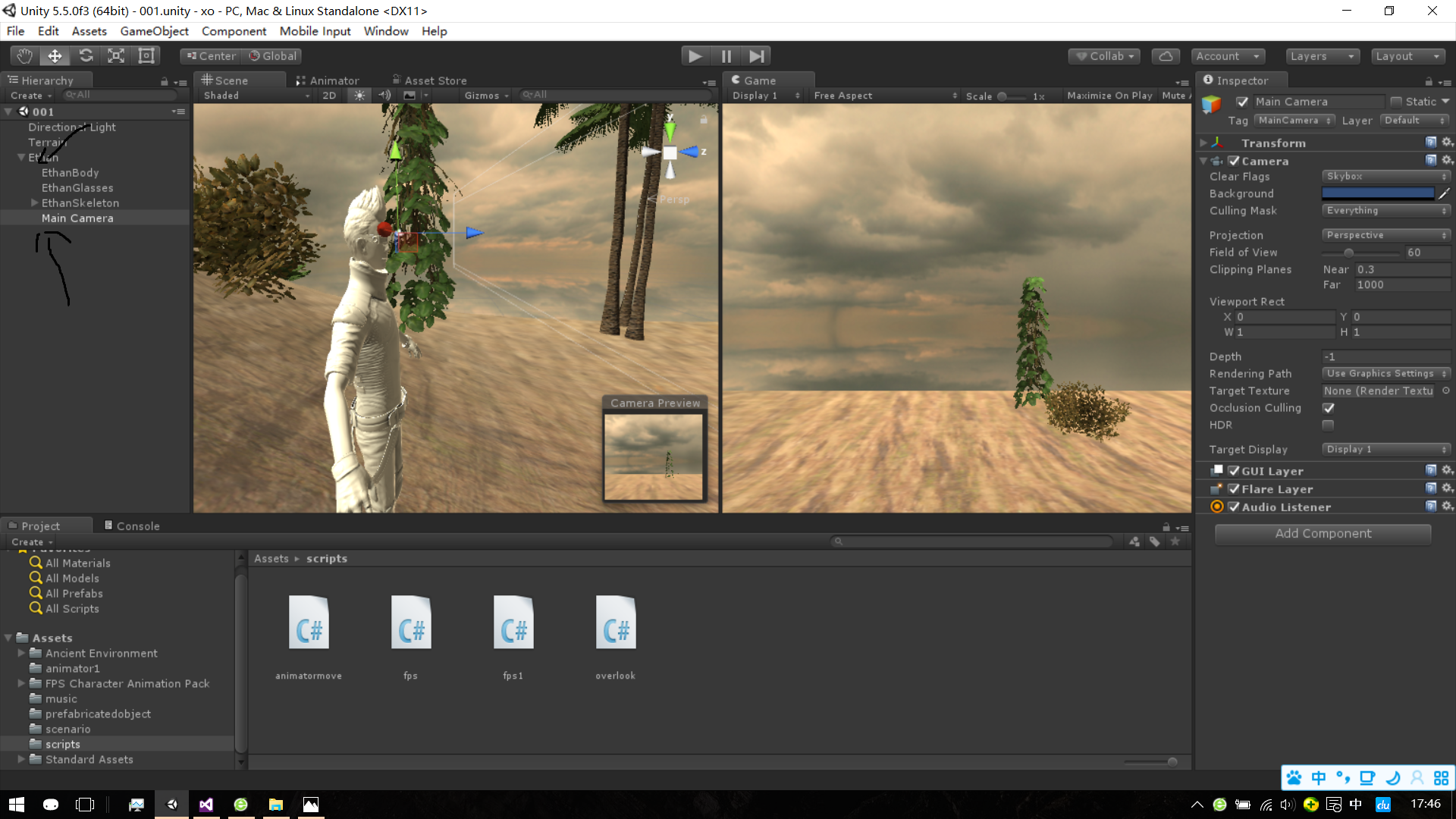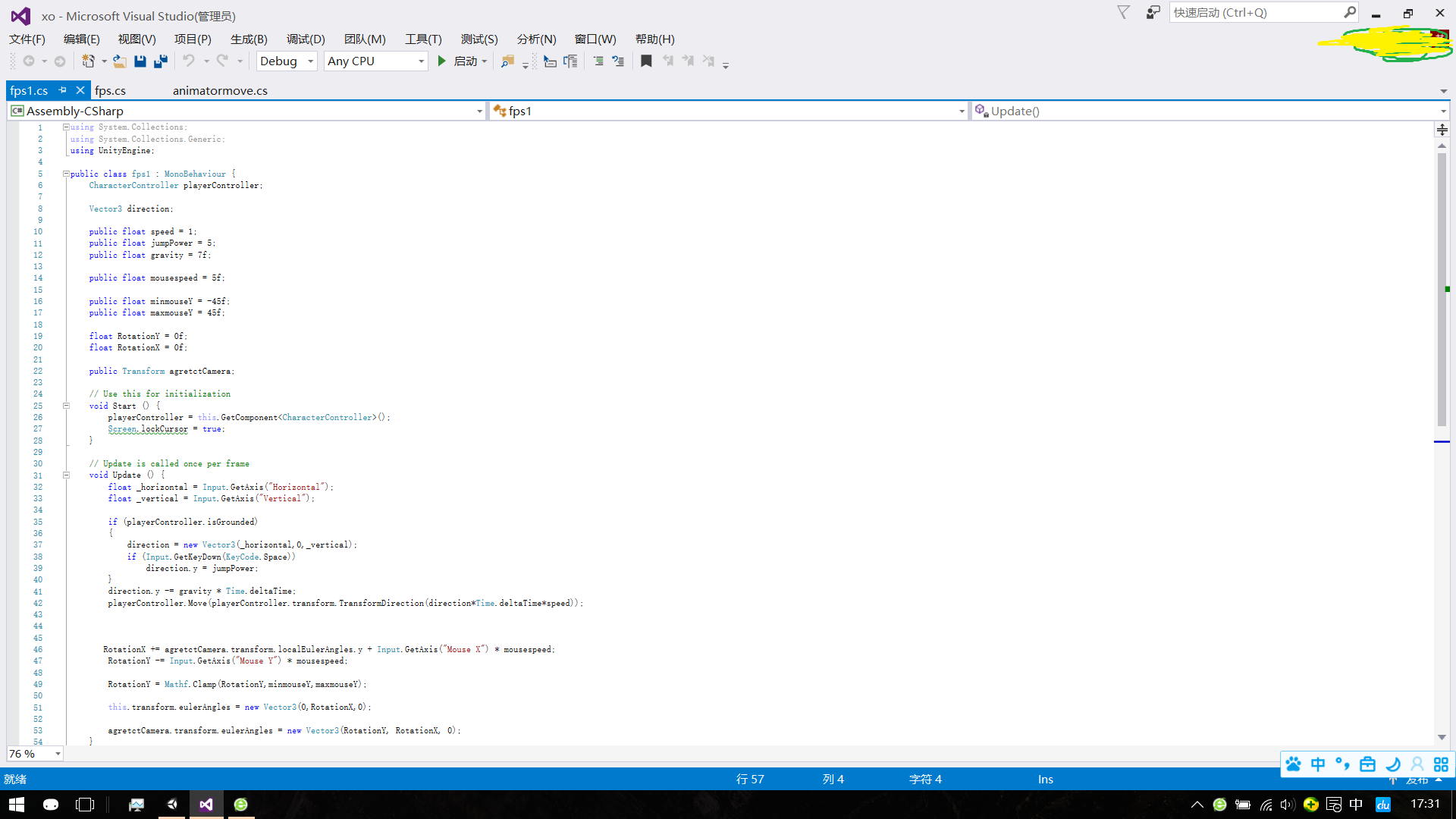• 第三人称是视角意味着摄像机跟随着角色移动而不是在角色内移动，模拟阴影，像实时阴影和光照贴图，改善图像。第三人称游戏
• UNITY 第三人称 人物移动代码详解 UNITY 第三人称 人物移动代码详解UNITY 人物移动
• unity制作第三人称移动控制脚本一个简单的第三人称角色移动脚本>>废话不多说，直接上代码新的改变功能快捷键合理的创建标题，有助于目录的生成如何改变文本的样式插入链接与图片如何插入一段漂亮的代码片生成...

一个简单的第三人称角色移动脚本>>

这里面的镜头控制我是用了unity自带的镜头插件Cinemachine，打开window->PackageManager，在All packages下找。建一个简单的角色，添加初始镜头。此时，点击运行，就可以通过鼠标控制镜头视角。不过效果并不好，接下来调整摄像机的数据。

1. 相机的活动范围2. 让角色动起来
首先在新建的角色上添加组件CharacterController。然后添加一个脚本命名为ThirdPerson（名字自定义）。ThirdPerson脚本代码：

/**
这里是通过角色控制器CharacterController来控制角色的移动
**/
public CharacterController P_controller;
public Transform N_camera;
public float P_speed = 6f;//角色移动速度
public float N_trunSnoothTime = 0.1f;//角色转身缓冲时间
float N_trunSmoothVelocity;
private void Update()
{
float N_horizontal = Input.GetAxisRaw("Horizontal");
float N_vertical = Input.GetAxisRaw("Vertical");

Vector3 P_direction = new Vector3(N_horizontal, 0, N_vertical).normalized;

if (P_direction.magnitude >= 0.1f) {
float N_targetAngle = Mathf.Atan2(P_direction.x, P_direction.z) * Mathf.Rad2Deg+N_camera.eulerAngles.y;
float N_angle = Mathf.SmoothDampAngle(transform.eulerAngles.y, N_targetAngle, ref N_trunSmoothVelocity, N_trunSnoothTime);
transform.rotation = Quaternion.Euler(0f, N_angle, 0f);

Vector3 N_moveDir = Quaternion.Euler(0f, N_targetAngle, 0f) * Vector3.forward;
P_controller.Move(N_moveDir.normalized * P_speed * Time.deltaTime);
}
}

写好代码保存返回unity，将CharacterController组件和主摄像机拖拽到相应的位置：1. 最后优化：给摄像机添加碰撞检测，目的是防止有遮挡物挡住镜头看不到角色到此，第三人称移动控制算是完成了。我是Pex-叶巴适！一只小菜鸟。这算是我学习的笔记，可能有不足之处，可以在下方留言，大家一起学习！^_^。

展开全文unity c# 游戏开发
• 人称视角,旋转,缩放,移动,跳跃,人称视角,旋转,缩放,移动,跳跃,人称视角,旋转,缩放,移动,跳跃,人称视角,旋转,缩放,移动,跳跃,人称视角,旋转,缩放,移动,跳跃,人称视角,旋转,缩放,移动,跳跃,第一人称视角
• Unity第三人称人物移动

千次阅读 2020-09-05 21:29:53
要实现第三人称的人物移动首先就是需要控制人物的移动： 1.创建一个简单的物体当作人物： 2，为物体添加移动代码：本次使用的方式A、D键控制前后运动，W、S键控制转向 transform.Translate(0, 0, speed * Input....

1，创建一个简单的物体作为角色2，为物体添加移动代码：本次使用的方式A、D键控制前后运动，W、S键控制转向

transform.Translate(0, 0, speed * Input.GetAxis("Vertical"));//Translate可以控制物体移动
transform.Rotate(0, speed *20* Input.GetAxis("Horizontal"),0);//Rotate可以控制物体转向

if(Input.GetKeyDown(KeyCode.Space))
{
}

4，为了使得物体不摔倒，使用eulerAngles给物体一个角度判断

if ((this.transform.eulerAngles.x > 90 && this.transform.eulerAngles.x < 270) || (this.transform.eulerAngles.z > 90 && this.transform.eulerAngles.z < 270))
{
transform.eulerAngles = new Vector3(0, 180, 0);
}

5，给物体添加一个跟随相机，首先创建一个相机，然后把这个脚本赋给相机，player为玩家，通过玩家的位置，来为相机创建一个跟随位置。
第二行的lerp是一个插值运算方法，可以使得相机平滑的跟随着人物来运动。
为了使得相机在人物背后，可以使用LookAt来注视玩家。

Vector3 camera_felow = player.position + Vector3.up * speed_one - first.forward * speed_two;
transform.position = Vector3.Lerp(transform.position, camera_felow, Time.deltaTime * smooth);
transform.LookAt(first.position);//lookat为注视物体的效果

最后附上完整的两个代码：
玩家：脚本直接添加在需要控制移动的角色身上

using System.Collections;
using System.Collections.Generic;
using UnityEngine;

public class three_run : MonoBehaviour
{
public  float speed = 0.1f; //定义一个速度
void Update()
{
//控制物体的移动
transform.Translate(0, 0, speed * Input.GetAxis("Vertical"));
transform.Rotate(0, speed *20* Input.GetAxis("Horizontal"),0);
//实现跳跃的效果
if(Input.GetKeyDown(KeyCode.Space))
{
}
//防止物体倒下（如果是角色控制器可以直接面板调整）
if ((this.transform.eulerAngles.x > 90 && this.transform.eulerAngles.x < 270) || (this.transform.eulerAngles.z > 90 && this.transform.eulerAngles.z < 270))
{
transform.eulerAngles = new Vector3(0, 180, 0);
}
}
}

相机：脚本添加到相机上面， 并将跟随的角色拖入

using System.Collections;
using System.Collections.Generic;
using UnityEngine;

public class three_camera : MonoBehaviour
{
public Transform player;
public float speed_one=10f;
public float speed_two=10f; //使用speed_one,speed_two可以调整玩家和相机的距离
private float smooth = 1f;
void LateUpdate()
{
Vector3 camera_felow = player.position + Vector3.up * speed_one - player.forward * speed_two;
transform.position = Vector3.Lerp(transform.position, camera_felow, Time.deltaTime * smooth);
transform.LookAt(player.position);
}
}

展开全文unity unity3d 游戏开发
• 单指旋转镜头，同时摇杆控制移动 双指缩放镜头 镜头旋转不等低于人脚下 镜头与人连线中间碰墙，拉进镜头 镜头控制 using UnityEngine; using System.Collections; using UnityEngine.EventSystems; using System....

主要功能

1. 单指旋转镜头，同时摇杆控制移动
2. 双指缩放镜头
3. 镜头旋转不等低于人脚下
4. 镜头与人连线中间碰墙，拉进镜头

镜头控制

using UnityEngine;
using System.Collections;
using UnityEngine.EventSystems;
using System.Collections.Generic;

public class MouseOrbitImproved : MonoBehaviour
{
public bool m_isScale = true;
public Transform target;
public float distance = 8.0f;
public float xSpeed = 70.0f;
public float ySpeed = 50.0f;

public float yMinLimit = 0f;
public float yMaxLimit = 90f;

public float distanceMin = 8f;
public float distanceMax = 15f;
public float zoomSpeed = 0.5f;

private float x = 0.0f;
private float y = 0.0f;

private float fx = 0f;
private float fy = 0f;
private float fDistance = 0;

int m_fingerId = -1; //  当摇杆移动，控制镜头的手指
bool m_isClickUi = false;
Dictionary<int, bool> m_dicTouch = new Dictionary<int, bool>(); //key 为fingerid ，value为是否接触到UI
Transform m_trans;
float t = 0.2f;
public float m_minDis = 1.0f;
public bool m_isNear = false;
// Use this for initialization
void Start()
{
m_trans = this.transform;
Vector3 angles = transform.eulerAngles;
x = angles.y;
y = angles.x;
fx = x;
fy = y;

UpdateRotaAndPos();
fDistance = distance;
}

void Update()
{

if (Input.touchCount == 0)//当前没有手指,全部初始化
{
m_fingerId = -1;
m_dicTouch.Clear();
}

if (Input.touchCount == 1)
{
List<int> deleteFinger = new List<int>();
foreach (var item in m_dicTouch)
{
if (item.Key != Input.GetTouch(0).fingerId)
{
}
}

//删除m_dicTouch非当前单指的item项目--》得到结果就是m_dicTouch只有当前单指或者啥也没有
for (int i = 0; i < deleteFinger.Count; i++)
{
//Debug.Log("单指：删除：" + deleteFinger[i]);
m_dicTouch.Remove(deleteFinger[i]);
}

switch (Input.GetTouch(0).phase)
{
case TouchPhase.Began:
if (EventSystem.current.IsPointerOverGameObject(Input.GetTouch(0).fingerId))
{
//Debug.Log("单指：增加true：" + Input.GetTouch(0).fingerId);
m_dicTouch[Input.GetTouch(0).fingerId] = true; //如果按在ui上为true
}
else
{
//Debug.Log("单指：增加false：" + Input.GetTouch(0).fingerId);
m_dicTouch[Input.GetTouch(0).fingerId] = false;
}

break;
case TouchPhase.Moved:

break;
case TouchPhase.Stationary:
break;
case TouchPhase.Ended:
break;
case TouchPhase.Canceled:
break;
default:
break;
}

}

if (Input.touchCount == 2)
{
List<int> deleteFinger = new List<int>();

foreach (var item in m_dicTouch)
{
bool isDelete = true;
for (int i = 0; i < Input.touchCount; i++)
{
if (item.Key == Input.touches[i].fingerId)
{
isDelete = false;
break;
}
}
if (isDelete == true)
{
}
}

//删除m_dicTouch里面非现在按下的两个手指--》得到的结果：m_dicTouch里有一个现在按下手指中的0-2个
for (int i = 0; i < deleteFinger.Count; i++)
{
//Debug.Log("双指：删除：" + deleteFinger[i]);
m_dicTouch.Remove(deleteFinger[i]);
}

for (int i = 0; i < Input.touchCount; i++)
{
switch (Input.touches[i].phase)
{
case TouchPhase.Began:
if (EventSystem.current.IsPointerOverGameObject(Input.touches[i].fingerId))
{
//Debug.Log("双指：增加true：" + Input.touches[i].fingerId);
m_dicTouch[Input.touches[i].fingerId] = true;

}
else
{
//Debug.Log("双指：增加false：" + Input.touches[i].fingerId);
m_dicTouch[Input.touches[i].fingerId] = false;
}
break;
case TouchPhase.Moved:
break;
case TouchPhase.Stationary:
break;
case TouchPhase.Ended:
break;
case TouchPhase.Canceled:
break;
default:
break;
}
}

if (IsCanScale() == true && m_isScale == true)
{
Touch touchZero = Input.GetTouch(0);
Touch touchOne = Input.GetTouch(1);

// 上一帧每个点位置
Vector2 touchZeroPrevPos = touchZero.position - touchZero.deltaPosition;
Vector2 touchOnePrevPos = touchOne.position - touchOne.deltaPosition;

//上一帧两点距离的平方，不要做平方根运算，消耗大
float prevTouchDeltaMag = (touchZeroPrevPos - touchOnePrevPos).magnitude;
//这一帧两点距离的平方
float touchDeltaMag = (touchZero.position - touchOne.position).magnitude;

// Find the difference in the distances between each frame.
float deltaMagnitudeDiff = prevTouchDeltaMag - touchDeltaMag;
fDistance = Mathf.Clamp(distance + deltaMagnitudeDiff * zoomSpeed, distanceMin, distanceMax);
}

}

if (Application.isMobilePlatform == false && m_isScale == true)
{
if (Input.GetAxis("Mouse ScrollWheel") > 0)
{
fDistance = Mathf.Clamp(distance - zoomSpeed, distanceMin, distanceMax);
}
else if (Input.GetAxis("Mouse ScrollWheel") < 0)
{
fDistance = Mathf.Clamp(distance + zoomSpeed, distanceMin, distanceMax);
}
}

distance = Mathf.Lerp(distance, fDistance, 0.25f);
}

void LateUpdate()
{
if (Application.isMobilePlatform)
{
if (Input.GetMouseButton(0) && IsCanRotate())
{
Touch input = new Touch();
for (int i = 0; i < Input.touchCount; i++)
{
if (Input.touches[i].fingerId == m_fingerId)
{
input = Input.touches[i];//找到控制镜头移动的手指
}
}
if (target)
{
float dx = Input.GetAxis("Mouse X");
float dy = Input.GetAxis("Mouse Y");
if (Input.touchCount > 0)
{
dx = input.deltaPosition.x;
dy = input.deltaPosition.y;
}

x += dx * xSpeed * Time.deltaTime;//*distance
y -= dy * ySpeed * Time.deltaTime;

y = ClampAngle(y, yMinLimit, yMaxLimit);
}
}
}
else
{
if (Input.GetMouseButtonDown(0))
{
if (EventSystem.current.IsPointerOverGameObject())
{
m_isClickUi = true;
}
}

if (Input.GetMouseButtonUp(0))
{
m_isClickUi = false;
}
if (m_isClickUi == false && Input.GetMouseButton(0))
{
if (target)
{
float dx = Input.GetAxis("Mouse X");
float dy = Input.GetAxis("Mouse Y");

x += dx * xSpeed * 20 * Time.deltaTime;//*distance
y -= dy * ySpeed * 20* Time.deltaTime;

y = ClampAngle(y, yMinLimit, yMaxLimit);
}
}

}

fx = Mathf.Lerp(fx, x, 0.2f);
fy = Mathf.Lerp(fy, y, 0.2f);

UpdateRotaAndPos();
}

bool m_isLastHit = false;
void UpdateRotaAndPos()
{
if (target)
{
bool isCurHit = false;
Quaternion rotation = Quaternion.Euler(fy, fx, 0);
Vector3 negDistance = new Vector3(0.0f, 0.0f, -distance);
Vector3 position = rotation * negDistance + target.position; //摄像头的位置为 移动后角度*距离 + 目标位置

Vector3 canSetPos = position;

//m_trans.position = position;

//Debug.DrawLine(target.position, m_trans.position, Color.red);
//主角朝着这个方向发射射线
RaycastHit hit;

if (Physics.Linecast(target.position, position, out hit))
{
Debug.Log("摄像机碰到:" + hit.collider.gameObject);

//当碰撞的不是摄像机也不是地形 那么直接移动摄像机的坐标
Vector3 posHit = hit.point;
Vector3 dir = posHit - target.position;
//Vector3 posEnd = posHit - dir * 0.5f;
canSetPos = posHit - dir * 0.5f;
isCurHit = true;
//m_trans.position = posEnd;
//m_trans.position = position;
}

//摄像机与地面的检测，不要穿过地
if (canSetPos.y < target.parent.position.y)
{
Debug.Log("摄像机碰到了地");
canSetPos.y = target.parent.position.y;

Vector3 relativePos = target.position - canSetPos;
rotation = Quaternion.LookRotation(relativePos);
isCurHit = true;
}

//float oldDis = (m_trans.position - target.position).sqrMagnitude;

//float newDis = (canSetPos - target.position).sqrMagnitude;

//if ((isCurHit == false && m_isLastHit == false) || (isCurHit == true && m_isLastHit == false) || (isCurHit == true && m_isLastHit == true)) //拉进是突进
//{
//    m_trans.position = canSetPos;
//}
//else if (m_isLastHit == true && isCurHit == false)
//{
//    Debug.Log("镜头远离墙体");
//    Vector3 mainCamPos = m_trans.position;
//    Vector3 newPos = Vector3.Lerp(mainCamPos, canSetPos, getCurrentDeltaTime() * movementLerpSpeed);
//    m_trans.position = newPos;
//}

m_trans.position = canSetPos;
m_trans.rotation = rotation;
m_isLastHit = isCurHit;
}
}
float movementLerpSpeed =0.1f;
float currentDeltaTime;
public float getCurrentDeltaTime()
{
currentDeltaTime = Time.deltaTime;

return currentDeltaTime;
}

public static float ClampAngle(float angle, float min, float max)
{
if (angle < -360F)
angle += 360F;
if (angle > 360F)
angle -= 360F;
return Mathf.Clamp(angle, min, max);
}

/// <summary>
/// 是否可以选择
/// </summary>
/// <returns></returns>
bool IsCanRotate()
{
bool ret = false;
if (m_dicTouch.Count == 1) //只有一个手指按下，并且没按在UI上
{
foreach (var item in m_dicTouch)
{
if (item.Value == false)
{
ret = true;
m_fingerId = item.Key;
}
}
}
else {
//当有两个手指按下，一个手指在UI（包含在UI摇杆）上，一个手指没在，可以移动镜头
int inUI = 0;
int outUI = 0;
foreach (var item in m_dicTouch)
{
if (item.Value == true)
{
inUI++;
}
else {
outUI++;
m_fingerId = item.Key;
}
}
if (inUI == 1 && outUI == 1)
{
ret = true;
}
}

return ret;
}

//何时才能双指控制缩放-->两个手指都没按在UI上
bool IsCanScale()
{
bool ret = true;
if (m_dicTouch.Count == 2)
{
foreach (var item in m_dicTouch)
{
if (item.Value == true)
{
ret = false;
break;
}
}
}
return ret;
}
}

解决手指a移动摇杆，手指b旋转镜头

1. 当同时按下两个手指，手指a按在UI上，手指b没按在UI上，为可以控制镜头旋转
2. 当只有一个手指按下，没按在UI上，可以控制镜头旋转
3. m_dicTouch中维护手指的状态，在双指按下是，找到没按在UI上的手指b的fingerId为控制参数

双指缩放镜头

两个手指都没按在UI上，取这一帧与上一帧的距离的平方差值，不能取平方根插值，多了性能消耗

人物朝向镜头正方向移动

void Update () {

//h = Input.GetAxis("Horizontal");
//v = Input.GetAxis("Vertical");
if (m_joy != null)
{
h = m_joy.Horizontal;
v = m_joy.Vertical;
moveVec = new Vector3(h, 0, v);

if (h != 0 || v != 0)
{
m_isJoyCtrl = true;
nav.ResetPath();
ani.SetBool("IsRun", true);
// 根据摄像机方向 进行移动
moveVec = Quaternion.Euler(0, m_camera.eulerAngles.y, 0) * moveVec;
nav.Move(moveVec.normalized * Time.deltaTime * m_speed);
RotatePlayer();
}

源码
https://github.com/luoyikun/VirtualCity
TestJoystick场景

展开全文unity3d 第三人称镜头控制 双指缩放
• 动手实现了一个人称视角的编写方式，以前一直都用的unity自带的standardassets里面的人称视角预制体，现在也能自己写出来这种视角了，根据里面的文档来写每个人都可以做到，文档还介绍了一些简单的知识点，...
• Unity3D FPS 第一人称视角移动

万次阅读 多人点赞 2018-01-17 19:54:30
一次写博客，有什么不足之处请多多原谅，本章的主要讲的是fps的人称视角，我们要知道，人称视角在unity3d里怎么实现，主要有两个，一个是主角和另一个是摄像机。 （如果你们认真看完这个教程fps人称...

第一次写博客，有什么不足之处请多多原谅，本章的主要讲的是fps的第一人称视角，我们要知道，第一人称视角在unity3d里怎么实现，主要有两个，一个是主角和另一个是摄像机。

（如果你们认真看完这个教程fps第一人称视角是完全可以学会的。不要跳行看，看完这个教程一个小时都不到。我相信有人连这个都没看直接看下面

首先上图：Ethan这个主角是unity自带的模型，Main Camera是一个摄像机。（图片我没处理（你以为我不想啊博客没有截屏我有ps但是我已经懒得处理了）自已放大看）

第一步：将摄像机移到主角身上作为一个子级物体（然后在Scene面板，将移动摄像机到主角面前如我上面那图）。如我上图那样子。（这时候陈独秀同学会问：上面图那么大你要我怎么找啊）陈独秀你给我过来我一巴掌你，你不会看Create面板吗？就是那个黑色箭头那个面板。对你没看错。就是黑色箭头那里。（虽然画的丑了点，但是你们看明白就行了）

然后可以写（复制）代码了是不是很开心。（开心个鬼看到代码就直接复制粘贴你们nb，不修改，你们复制下面的代码能运行我拜你为师）（照着下面照片的代码抄的同学注意了最后面一行还有一个}）对没错就是上面那个那些代码。就50行不到就ok了，完成第一人称视角。（陈独秀：解析呢。都没有解析学个毛啊）

唉陈独秀同学就是这么心急。我一行一行给你们解析清楚ok(说是这么说反正你们又不顺着网线来打我)；

ps： 别复制下面代码。

CharacterController playerController;  //这行代码是声明一个CharacterController类；

Vector3 direction; //声明3维向量；

public float speed = 1;   //主角的移动速度
public float jumpPower = 5; //主角跳的高度
public float gravity = 7f; //重力

public float mousespeed = 5f; //鼠标快慢

public float minmouseY = -45f; //限制鼠标y轴（你们想一想眼睛能看到后面的吗？可以的话你nb）
public float maxmouseY = 45f;

float RotationY = 0f;
float RotationX = 0f;这两个存放的是鼠标输入

public Transform agretctCamera;  //这个必须在Create面板拖放Main Camera（也就是摄像机）这个位置里否则没有效果，这个类是存放的是摄像机位置；

// Use this for initialization
void Start () {

//这个函数就不说明了，看到这里的都是基础过关了吧？
playerController = this.GetComponent<CharacterController>(); //获取依附在这个物体上的CharacterController组件

Screen.lockCursor = true;//鼠标隐藏（新的unity5.0版本以上好像用这个会警告，我就不用新的了反正旧的能用我去研究新的用法干嘛，我有病啊，（你以为我会告诉你我他喵的根本看不懂官方怎么解析的么呵呵））

}

// Update is called once per frame
void Update () {//这个方法都要我解析，陈独秀你过来，（蠢蠢欲动的陈独秀：我什么都没说好吗，关我什么事）

float _horizontal = Input.GetAxis("Horizontal");//将水平轴的给_horizontal
float _vertical = Input.GetAxis("Vertical");//同上（呵呵我会告诉你我懒得打）

if (playerController.isGrounded)
//（陈独秀：这个playerController是什么鬼啊）呵呵陈独秀同学坐下，我先给你一巴掌先前面不是声明了playerController获取CharacterController组件吗？这么快就忘了 你很棒棒噢。CharacterController组件中有一个检测是否到地面的方法就是.isGrounded。这个在CharacterController才有的，记住了陈独秀同学。playerController.isGrounded也就是说主角在地面上。
{
direction = new Vector3(_horizontal,0,_vertical);//在地面上才能移动，这个是移动向量

if (Input.GetKeyDown(KeyCode.Space))     //检测你是否按下了空格键
direction.y = jumpPower;      //如果按下了空格键就三维向量中的y轴等于jumpPower（呵呵，陈独秀同学是不是又忘了jumpPower是什么了，回头看看都声明了什么）
}
direction.y -= gravity * Time.deltaTime;//这个在if语句外也就是说主角的向下的重力

playerController.Move(playerController.transform.TransformDirection(direction*Time.deltaTime*speed));
//这句就有点难理解了，我都不知道怎么解析， playerController.Move（）这个是移动方法，playerController.transform.TransformDirection(）这个是跟随世界坐标的意思，如果没有这个主角身体转向的时候它就是一个方向，按w的时候永远只朝着一个方向移动。唉解析什么的最烦了自已百度。

RotationX += agretctCamera.transform.localEulerAngles.y + Input.GetAxis("Mouse X") * mousespeed;

//存放摄像机（也就是鼠标）x轴
RotationY -= Input.GetAxis("Mouse Y") * mousespeed;//存放摄像机（也就是鼠标）y轴

RotationY = Mathf.Clamp(RotationY,minmouseY,maxmouseY);//限制鼠标上下，Mathf这个是数学函数的意思，Math不是数学吗？f不就是初中，高中的时候  你们最讨厌的函数么，像 f=xxx+xx+x那种；

this.transform.eulerAngles = new Vector3(0,RotationX,0);//主角的y轴旋转（为什么有两个0，你想想主角围着x轴旋转会怎样）

agretctCamera.transform.eulerAngles = new Vector3(RotationY, RotationX, 0);//这个是摄像机上下左右。

好了就到这了，有些自已百度吧，比如eulerAngles这个是什么意思（难道还要我解析给你们看，我又不是百科全书，想学的人我不说也会，不想学的他早就xx掉我这条博客了，反之他又看不到，）重要的是自已理解。不是复制代码，没有用的。最后保存上面代码图片自已手动打一遍吧。不会在下面留评论，别乱喷人啊，我幼小的心灵很脆弱的，（四年前的憨憨语言）

最后给上源码一份。

这个代码可以复制哦：（照着上面图片抄的同学后悔了没）  （我回来了，时隔4年，我看到有很多人都来看这个，有什么想学的，可以在评论或者私信我，有时间我会出一个教程）

using System.Collections;
using System.Collections.Generic;
using UnityEngine;

public class fps1 : MonoBehaviour {
CharacterController playerController;

Vector3 direction;

public float speed = 1;
public float jumpPower = 5;
public float gravity = 7f;

public float mousespeed = 5f;

public float minmouseY = -45f;
public float maxmouseY = 45f;

float RotationY = 0f;
float RotationX = 0f;

public Transform agretctCamera;

// Use this for initialization
void Start () {
playerController = this.GetComponent<CharacterController>();
Screen.lockCursor = true;
}

// Update is called once per frame
void Update () {
float _horizontal = Input.GetAxis("Horizontal");
float _vertical = Input.GetAxis("Vertical");

if (playerController.isGrounded)
{
direction = new Vector3(_horizontal,0,_vertical);
if (Input.GetKeyDown(KeyCode.Space))
direction.y = jumpPower;
}
direction.y -= gravity * Time.deltaTime;
playerController.Move(playerController.transform.TransformDirection(direction*Time.deltaTime*speed));

RotationX += agretctCamera.transform.localEulerAngles.y + Input.GetAxis("Mouse X") * mousespeed;
RotationY -= Input.GetAxis("Mouse Y") * mousespeed;
RotationY = Mathf.Clamp(RotationY,minmouseY,maxmouseY);
this.transform.eulerAngles = new Vector3(0,RotationX,0);
agretctCamera.transform.eulerAngles = new Vector3(RotationY, RotationX, 0);
}

}

展开全文unity3d
• 第三人称的角色移动控制方案有很多种： 1. rigidbody.velocity() : 通过控制刚体的速度 来实现对角色的移动控制 2. rigidbody.MovePosition() : 通过调整刚体的Position 来实现对角色的移动控制 3. rigidbody....Unity 游戏开发 角色控制
• private Vector3 moveDirection = Vector3.zero; private bool grounded = false; void FixedUpdate() { if (grounded) { if (!(Input.GetKey(KeyCode.LeftControl)||Input.GetKey(KeyCode.RightControl))) //如果...
• } private void MovementSolution3(float h, float v) { Vector3 movement = new Vector3(h, 0f, v); movement = movement.normalized * speed * Time.fixedDeltaTime; transform.Translate(movement); } private ...unity3d
•  //获取相机移动的高度  float m_wangtedHeight = m_player.transform.position.y + m_distanceUp;  //获得相机当前角度  float m_currentRotationAngle = m_transsform.eulerAngles.y;  //获取相机当前的...unity3d
• 第三人称根据相机控制去旋转与移动 using System.Collections; using System.Collections.Generic; using UnityEngine; public class PlayerController : MonoBehaviour { private Animator anim; private ...
• 在Capsule下增加Character Controller，新增FPLook.cs（鼠标控制的人称视角）和FPMove.cs（键盘WSAD控制的前后左右移动）： FPLook.cs： using System.Collections; using System.Collections.Generic; ...c#
• Unity3D第三人称摄像机

千次阅读 2018-10-17 16:40:34
创建一个名为CameraBase的空物体，把该物体移动至人物模型的上半身的中央处，世界坐标为（0, 1.35, 0），这个物体相当于是摄像机的基准点。随后我把摄像机设为CameraBase的子物体，把摄像机拖到人物模型的后方，...
• 需要物体：一个用于移动的对象，一个主摄像机，一个地面对象 摄像机的父结点为移动对象 旋转移动坐标示意图: 用于视角移动的代码: using System.Collections; using System.Collections.Generic; using ...Unity3D
• 这个Demo主要是针对Unity3D第三人称视角模型（相机跟随）的一个简单实现，记录一下实现的过程与核心代码。 Idea 在Unity3D中导入一个人物模型，并创建一个可供人物活动的Terrain 调整摄像机至正确的视角（...
• 一步：搭建场景 因为这个游戏只针对游戏逻辑为重点，所以搭建场景和人物素材我都从unity asset store中的免费资源下载：所以先找一个场景。 就用这个unity提供的免费的场景把 场景大概是这个样子的 上面的...unity3d 游戏开发
• /// /// 摄像机与player之间的方向向量 /// private Vector3 direction ; void Awake() { //获取到player的transform(Tags.Player是player的标签，如果有不明白什么意思的，可以看我前几篇文章，标签的管理) player...unity 游戏开发
• Unity 3D 如何实现人称视角 思路：人称的视角移动最关键的就是对摄像机的操作了吧。首先一个就是灵敏度的设置。其次就是转的速度。 public class xiangji : MonoBehaviour { private float x, y;//坐标x与y...unity3d
• 何谓第三人称？就像这样： 用wasd控制人物移动，同时保持在相机的中心。用鼠标右键与滚轮控制相机的角度和距离。 先说一下人物的移动： 首先给作为主角的单位加上 Charactor Controller组件，并调整胶囊型的...
• - 使用wasd控制人物的前后左右移动，空格发生跳跃，鼠标控制视线的移动 创建脚本MoveController1 using System.Collections; using System.Collections.Generic; using System.IO; using System.Threading; using ...unity3d
• Unity3D实战入门之第三人称射击游戏（TPS） 6年程序开发经验，精通C...
• Unity第三人称视角摄像机、跟随与球面旋转逻辑释义图解分析Unity C#代码 逻辑释义 【关键transform】 1[玩家] 2[注视目标点] 3[摄像机] [摄像机]跟随[注视目标点]，[注视目标点]跟随[玩家] 【看向注视目标点】 ...Unity C#
• 设置第三人称视角 方式一：（设置子对象） 1.将摄像机拖拽到合适的角度上 2.把摄像机拖拽进物体对象，摄像机成为物体的子对象，这样摄像机就可以跟着物体一起移动 方式二：（脚本设置） 创建摄像机...
• 效果： 项目包链接https://pan.baidu.com/s/1malCM72e3LcF4K4Ht_yMhA 提取码：syq1unity
• transform.localEulerAngles = new Vector3(_rotationX, _rotationY, 0); moveHor=input.getAxis("Horizontal"); moveVer=input.getAxis("Vertical"); transform.translate(moveHor,0.0f,moveVer,space.Self); }...
• 关于第三人称镜头和人物移动，我们的目标是实现一个玩家能够随意移动镜头观察环境，同时人物在静止时不会受到镜头方向影响，而移动时会受到影响的移动系统，就是比较常见的第三人称移动系统。 第一种实现方式，我...unity c#
• 4、注释第三人称的角色控制器脚本的关于移动的动画，否则与摇杆的动画结合混乱；另外，注释角色面向方位更新，避免移动操作，方位回到原来方向。 //更新角色控制器的移动面向方位（角色移动操作不是使用角色...unity3d
• 自学Unity3D有一段时间了，一直想弄一个第一人称角色控制器，网上还是有很多教程和资料，但感觉有很多教程和资料理解起来比较复杂，在这里我结合网上所学的知识自己写了一个比较容易理解的Unity3D第人称控制器脚本...unity3d unity 游戏开发 c#...